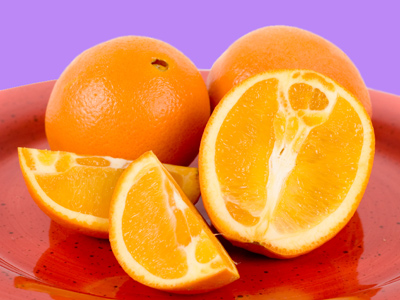24 is the same as 12 and 44 is the same as one whole.

# Year 2 Fractions - Equivalent Fractions

This quiz addresses the requirements of the National Curriculum KS1 Maths and Numeracy for children aged 6 and 7 in year 2. Specifically this quiz is aimed at the section dealing with recognising equivalent fractions.

Recognising, writing and naming fractions are all important steps in Year 2. Equivalent fractions means two or more fractions which have the same value. For example, two quarters and one half are both the same, as are two halves, four quarters and 1 whole.

For a full explanation of fractions, take a look at the Teaching Fractions page.

Question 1
Which pair of fractions have the same value?
23 and 12
14 and 24
44 and 22
13 and 24
Whenever the numerator and denominator are the same number, the fraction is equal to one whole
Question 2
Which is less: 14 of 12 or 13 of 9?
They both give the same answer
13 of 9
14 of 12
13 of 12
13 of 9 is 3, and 14 of 12 is also 3
Question 3
Which fraction is the same as 12?
34
14
25
24
There are four quarters in a whole, so two of them would be the same as a half
Question 4
Kim ate 14 of a pizza and Fran also ate 14. What fraction of the pizza remains?
14
34
13
12
There are four quarters in a whole. If two are eaten, two remain and this is the same as a half
Question 5
Which is more: 13 of 12 or 12 of 12?
They are the same
13 of 12
14 of 12
12 of 12
12 of 12 is 6 and 13 of 12 is 4
Question 6
If I cut a cake into four equal slices and eat two of them, how much have I eaten?
A third of the cake
A quarter of the cake
Half of the cake
Three quarters of the cake
Two quarters out of four is the same as one half
Question 7
Jim and Harry both have 12 jelly beans. Jim eats 14 of his and Harry eats 13 of his. Who ate more jelly beans?
Jim
Harry
They ate the same
Neither
14 of 12 is 3 and 13 of 12 is 4, so Harry ate one more than Jim
Question 8
Jane is given £24 for her birthday. She spends half the money on toys, a quarter of the money on sweets and gives a quarter to charity. How much money does Jane have left?
£2
£0
£1
£4
A half added to a quarter and another quarter equals everything!
Question 9
Which is less: 14 of 12 or 34 of 16?
34 of 16
24 of 16
14 of 12
14 of 16
34 of 16 is 12, 14 of 12 is only 3
Question 10
Which is more: 12 of 6 or 34 of 8?
34 of 8
12 of 6
They are the same
13 of 6
34 of 8 is 6, and 12 of 6 is 3